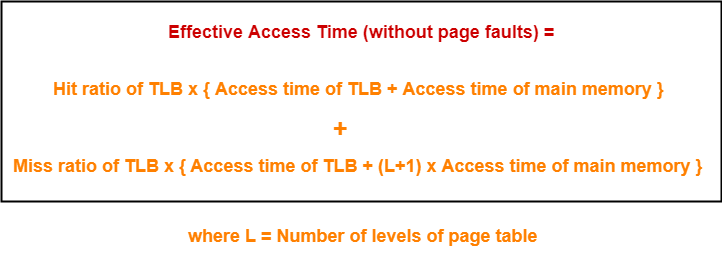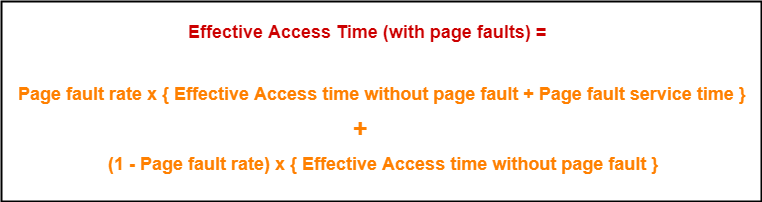# Page Fault | Paging | Practice Problems

## Page Fault in OS-

Before you go through this article, make sure that you have gone through the previous article on Page Fault in OS.

We have discussed-

• A page fault occurs when the referenced page is not found in the main memory.
• Page fault handling routine is executed on the occurrence of page fault.
• The time taken to service the page fault is called as page fault service time.

## Effective Access time-

In a multilevel paging scheme using TLB without any possibility of page fault, effective access time is given by-In a multilevel paging scheme using TLB with a possibility of page fault, effective access time is given by-## Problem-01:

Let the page fault service time be 10 ms in a computer with average memory access time being 20 ns. If one page fault is generated for every 106 memory accesses, what is the effective access time for the memory?

1. 21 ns
2. 30 ns
3. 23 ns
4. 35 ns

## Solution-

Given-

• Page fault service time = 10 ms
• Average memory access time = 20 ns
• One page fault occurs for every 106 memory accesses

### Page Fault Rate-

It is given that one page fault occurs for every 106 memory accesses.

Thus,

Page fault rate

= 1 / 106

= 10-6

### Effective Access Time With Page Fault-

It is given that effective memory access time without page fault = 20 ns.

Now, substituting values in the above formula, we get-

Effective access time with page fault

= 10-6 x { 20 ns + 10 ms } + ( 1 – 10-6 ) x { 20 ns }

= 10-6 x 10 ms + 20 ns

= 10-5 ms + 20 ns

= 10 ns + 20 ns

= 30 ns

Thus, Option (B) is correct.

## Problem-02:

Suppose the time to service a page fault is on the average 10 milliseconds, while a memory access takes 1 microsecond. Then, a 99.99% hit ratio results in average memory access time of-

1. 1.9999 milliseconds
2. 1 millisecond
3. 9.999 microseconds
4. 1.9999 microseconds
5. None of these

## Solution-

Given-

• Page fault service time = 10 msec
• Average memory access time = 1 μsec
• Hit ratio = 99.99% = 0.9999

Page fault rate

= 1 – Hit ratio

= 1 – 0.9999

= 0.0001

### Effective Access Time With Page Fault-

It is given that effective memory access time without page fault = 1 μsec.

Substituting values in the above formula, we get-

Effective access time with page fault

= 0.0001 x { 1 μsec + 10 msec } + 0.99999 x 1 μsec

= 0.0001 μsec + 0.001 msec + 0.9999 μsec

= 1 μsec + 0.001 msec

= 1 μsec + 1 μsec

= 2 μsec or 0.002 msec

Thus, Option (E) is correct.

## Problem-03:

If an instruction takes i microseconds and a page fault takes an additional j microseconds, the effective instruction time if on the average a page fault occurs every k instruction is-

1. i + j / k
2. i + j x k
3. (i + j) / k
4. (i + j) x k

## Solution-

Given-

• Page fault service time = j μsec
• Average memory access time = i μsec
• One page fault occurs every k instruction

### Page Fault Rate-

It is given that one page fault occurs every k instruction.

Thus,

Page fault rate

= 1 / k

### Effective Access Time With Page Fault-

It is given that effective memory access time without page fault = i μsec

Now, substituting values in the above formula, we get-

Effective access time with page fault

= (1 / k) x { i μsec + j μsec } + ( 1 – 1 / k) x { i μsec }

= j / k μsec + i μsec

= i + j / k μsec

Thus, Option (A) is correct.

## Problem-04:

Consider a system with a two-level paging scheme in which a regular memory access takes 150 nanoseconds and servicing a page fault takes 8 milliseconds. An average instruction takes 100 nanoseconds of CPU time and two memory accesses. The TLB hit ratio is 90% and the page fault rate is one in every 10,000 instructions. What is the effective average instruction execution time?

1. 645 nanoseconds
2. 1050 nanoseconds
3. 1215 nanoseconds
4. 1230 nanoseconds
5. None of these

## Solution-

Given-

• Number of levels of page table = 2
• Main memory access time = 150 ns
• Page fault service time = 8 msec
• Average instruction takes 100 ns of CPU time and 2 memory accesses
• TLB Hit ratio = 90% = 0.9
• Page fault rate = 1 / 104 = 10-4

Assume TLB access time = 0 since it is not given in the question.

Also, TLB access time is much less as compared to the memory access time.

### Effective Access Time Without Page Fault-

Substituting values in the above formula, we get-

Effective memory access time without page fault

= 0.9 x { 0 + 150 ns } + 0.1 x { 0 + (2+1) x 150 ns }

= { 0.9 x 150 ns } + { 0.1 x 450 ns }

= 135 ns + 45 ns

= 180 ns

### Effective Access Time With Page Fault-

Substituting values in the above formula, we get-

Effective access time with page fault

= 10-4 x { 180 ns + 8 msec } + (1 – 10-4) x 180 ns

= 8 x 10-4 msec + 180 ns

= 8 x 10-7 sec + 180 ns

= 800 ns + 180 ns

= 980 ns

### Effective Average Instruction Execution Time-

Effective Average Instruction Execution Time

= 100 ns + 2 x Effective memory access time with page fault

= 100 ns + 2 x 980 ns

= 100 ns + 1960 ns

= 2060 ns

Thus, Option (E) is correct.

## Problem-05:

A demand paging system takes 100 time units to service a page fault and 300 time units to replace a dirty page. Memory access time is 1 time unit. The probability of a page fault is p. In case of a page fault, the probability of page being dirty is also p. It is observed that the average access time is 3 time units. Then the value of p is-

1. 0.194
2. 0.233
3. 0.514
4. 0.981
5. None of these

## Solution-

Given-

• Page fault service time = 100 time units
• Time taken to replace dirty page = 300 time units
• Average memory access time = 1 time unit
• Page fault rate = p
• Probability of page being dirty = p
• Effective access time = 3 time units

Now, According to question-

3 time units = p x { 1 time unit + p x { 300 time units } + (1 – p) x { 100 time units } } + (1 – p) x { 1 time unit }

3 = p x { 1 + 300p + 100 – 100p } + (1 – p)

3 = p x { 101 + 200p } + (1 – p)

3 = 101p + 200p2 + 1 – p

3 = 100p + 200p2 + 1

200p2 + 100p – 2 = 0

On solving this quadratic equation, we get p = 0.019258

Thus, Option (E) is correct.

Get more notes and other study material of Operating System.

Watch video lectures by visiting our YouTube channel LearnVidFun.

SummaryArticle Name
Page Fault | Paging | Practice Problems
Description
Practice Problems based on Page Fault in OS. A page fault occurs when the referenced page is not found in the main memory. Effective access time is increased due to page fault service time.
Author
Publisher Name
Gate Vidyalay
Publisher Logo